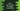# Java program to sort an array of integers in ascending order## Java program to sort an array of integers in ascending order :

In this Java programming tutorial, we will learn how to sort an array of integers in ascending order. Our program will first take the inputs from the user and create one integer array. Then it will sort the numbers of the array and print it out again to the user.

The size of the array is not fixed, i.e. the user will enter its size before entering the elements. Let’s take a look at the program :

### Java program :

``````import java.util.Scanner;

class Example {
public static void main(String[] args) {
// 1
Scanner sc = new Scanner(System.in);
int tempValue;

// 2
System.out.println("Enter number of array elements : ");
int size = sc.nextInt();

// 3
int[] numArray = new int[size];

// 4
for (int i = 0; i < size; i++) {
System.out.print("Enter element : ");
numArray[i] = sc.nextInt();
}

// 5
System.out.println("You have entered : ");
for (int i = 0; i < size - 1; i++) {
System.out.print(numArray[i] + ",");
}
System.out.println(numArray[numArray.length - 1]);

//6
for (int i = 0; i < numArray.length; i++) {
for (int j = i + 1; j < numArray.length; j++) {

//7
if (numArray[i] > numArray[j]) {
tempValue = numArray[i];
numArray[i] = numArray[j];
numArray[j] = tempValue;
}
}
}

//8
System.out.println("Final array after the sorting : ");
for (int i = 0; i < size - 1; i++) {
System.out.print(numArray[i] + ",");
}
System.out.println(numArray[numArray.length - 1]);

}
}``````

### Explanation :

The commented numbers in the above program denote the step number below :

1. Create one Scanner value sc to read the user input. Also, create one integer variable tempValue. We will use this integer variable for swapping two numbers below.
2. Ask the user to enter the size of the array. Read it and store it in variable size.
3. Create one integer array numArray. The size of the array is same as the user input size.
4. Using a for loop, take the values of all the elements of the array. Read each value and store it in the array.
5. Print out the entered values to the user. We are using one for loop here to read and print each element of the array.
6. This is the main step used for sorting the array. Here, we are running two for loops. The outer one will run from the first element to the last element, and the inner one will run from the current element pointed by the outer loop to the last element i.e., for each element, we are checking all elements to the right of it.
7. For an element pointed by the outer loop, if any element to the right of it is smaller than it, swap both elements. Here we are using the tempValue to temporarily store the value of one number.
8. Finally, print out the result to the user.

### Sample Output :

``````Enter number of array elements :
5
Enter element : 4
Enter element : 2
Enter element : 8
Enter element : 9
Enter element : 1
You have entered :
4,2,8,9,1
Final array after the sorting :
1,2,4,8,9``````

### Using Arrays.sort :

Instead of writing all of the sorting code, we can also use Arrays.sort() static method. This method takes one integer array as the parameter, and then it sorts all the elements of that array passed as the parameter.

The final java program will look like as below :

``````import java.util.Arrays;
import java.util.Scanner;

class Example {
public static void main(String[] args) {

Scanner sc = new Scanner(System.in);
int tempValue;

System.out.println("Enter number of array elements : ");
int size = sc.nextInt();

int[] numArray = new int[size];

for (int i = 0; i < size; i++) {
System.out.print("Enter element : ");
numArray[i] = sc.nextInt();
}

System.out.println("You have entered : ");
for (int i = 0; i < size - 1; i++) {
System.out.print(numArray[i] + ",");
}
System.out.println(numArray[numArray.length - 1]);

Arrays.sort(numArray);

System.out.println("Final array after the sorting : ");
for (int i = 0; i < size - 1; i++) {
System.out.print(numArray[i] + ",");
}
System.out.println(numArray[numArray.length - 1]);

}
}``````

Sample output :

``````Enter number of array elements :
7
Enter element : 1
Enter element : 4
Enter element : 3
Enter element : 9
Enter element : 10
Enter element : 2
Enter element : 33
You have entered :
1,4,3,9,10,2,33
Final array after the sorting :
1,2,3,4,9,10,33``````

As you can see, instead of writing down two for loops, we can use Arrays.sort to do this only in one line.